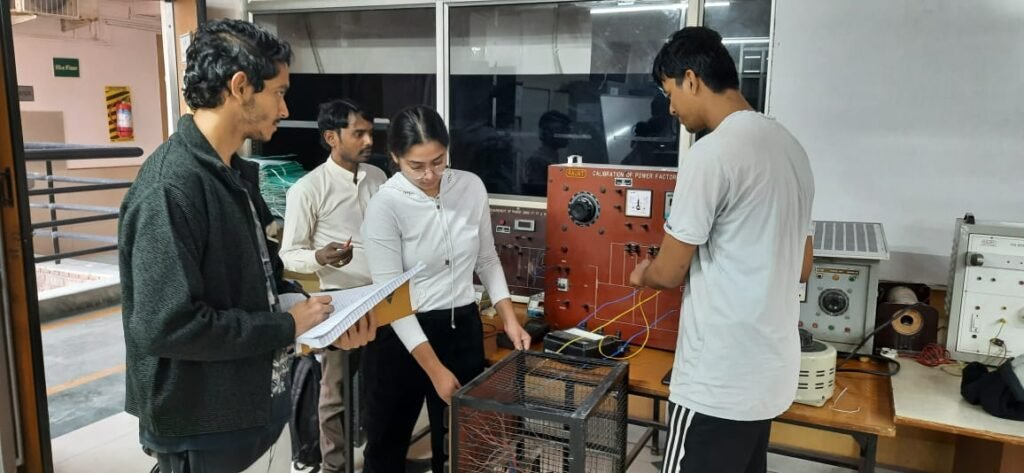Lab Room No: A307

Lab In charge: Mrs. SHASHI GANDHAR

Lab Attendant: Mr. Prem Singh

Lab Subjects:  UEEE LAB

In the UEE lab section of this course, the students learn to use detailed wiring diagrams to connect electric machines with power sources, loads, and meters to perform many different types of tests and experiments that demonstrate the operational and loading characteristics of electric machine systems. There is also a strong mathematical modeling and calculation aspect to this course as well. Students learn to conduct standard machine tests from which mathematical models for the machines can be determined empirically. Loading tests are then done on the machines, and the measured quantities from the loading tests are compared to quantities calculated mathematically by the students using machine models and circuit analysis techniques.Hardware Details: 1Phase Energy meter kits, polar curve & inverse law kits, Iron losses kits, power factor meter kits, Transformer turns Ratio kits, Phantom Loading kits.

Software Details: MATLAB

List of Experiments:

1. Preparation of Calibration Chart for 1-Phase Energy Meter
2. To plot polar curves for various lamps
3. To prove inverse square law of light
4. Measurement of Iron losses in a given bar specification
5. Preparation of Calibration Chart for Power Factor Meter.
6. Measurement of Transformer Turns Ratio.
7. Testing of Dielectric Strength of Transformer Oil.